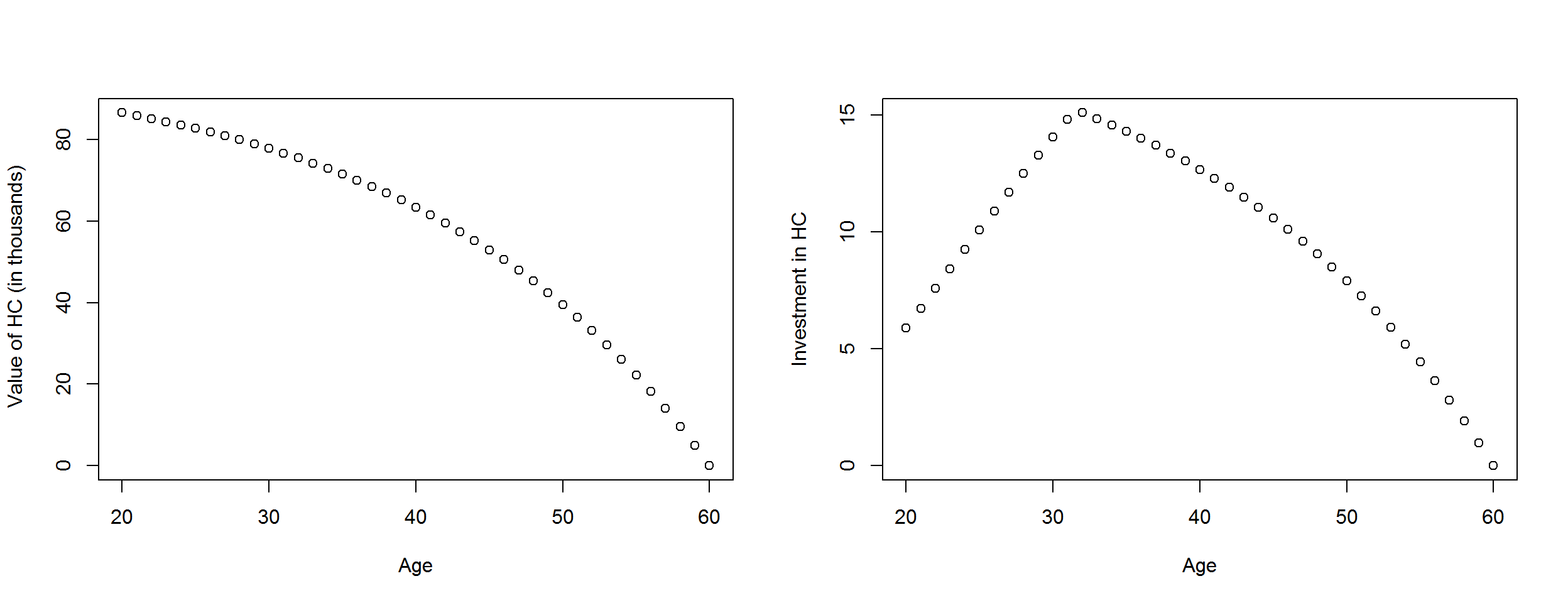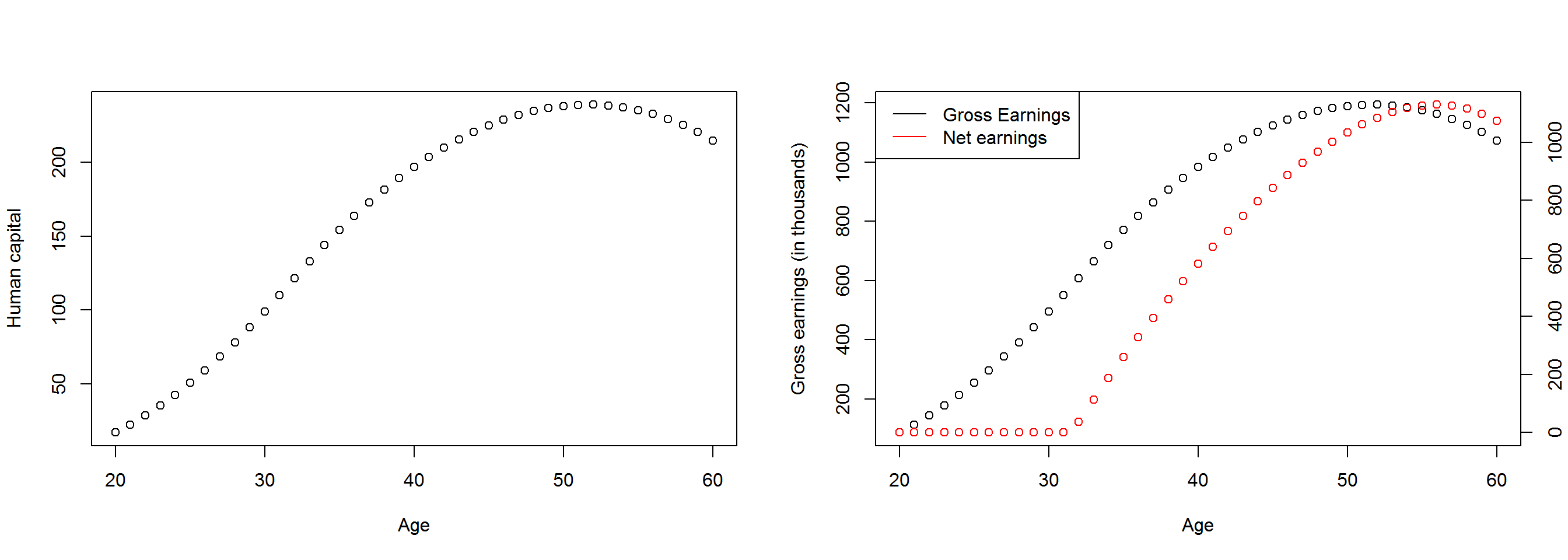## The Ben-Porath Model.

Assume the Human Capital Production Function is $$I_t= \sqrt(2) \left(\theta_t H_t \right)^{(1/2)}$$ where

• $$\theta_t$$ is the share of time spend on investing on human capital at t,

• $$H_t$$ is the human capital at t, and I_t represents new units of human capital produced at t. This unit of human capital can be used in the market for the first time in the next period. Assume that human capital depreciates at rate $$\delta$$ and individuals discount the future at rate r. Further assume that the rental rate per unit of human capital is w and constant over time.

Assume individuals work until they are 60 years old.

1. Show the exact expression of the value of a unit of human capital at age t, where t is assumed to be subsequent to schooling.All else equal, holding age fixed, the value of a unit of human capital is larger if an individual is still in school than if the individual has left schooling. Explain why.
• Value of human capital at age t \begin{align} V(age=60) &= 0 \\ V(age=59) &= \dfrac{1}{1+r} w_{60} \\ V(age=58) &= \dfrac{1}{1+r} \left[w_{59}+ \left(\dfrac{1-\delta}{1+r} \right) w_{60}\right] \\ V(age=57) &= \dfrac{1}{1+r} \left[ w_{58} + \left(\dfrac{1-\delta}{1+r} \right) w_{59}+\left(\dfrac{1-\delta}{1+r} \right)^2 w_{60}\right] \\ V(age=56) &= \dfrac{1}{1+r} \left[ w_{57} + \left(\dfrac{1-\delta}{1+r} \right) w_{58} + \left(\dfrac{1-\delta}{1+r} \right)^2 w_{59}+\left(\dfrac{1-\delta}{1+r} \right)^3 w_{60}\right] \\ . & \\ . & \\ V(age=t) &= \dfrac{1}{1+r} \left[ w_{t+1} + \left(\dfrac{1-\delta}{1+r} \right) w_{t+2}+\left(\dfrac{1-\delta}{1+r} \right)^2 w_{t+3} +... +\left(\dfrac{1-\delta}{1+r} \right)^{60-t-1} w_{60} \right] \end{align}
If the wage is constant over time : $$w_t=w$$ \begin{align} V(age=t) &= \dfrac{w}{1+r} \left[ 1 + \left(\dfrac{1-\delta}{1+r} \right) +\left(\dfrac{1-\delta}{1+r} \right)^2 +... +\left(\dfrac{1-\delta}{1+r} \right)^{60-t-1} \right] \\ & = \dfrac{w}{1+r} \left[ \dfrac{1- \left(\dfrac{1-\delta}{1+r} \right)^{60-t}}{1- \left(\dfrac{1-\delta}{1+r} \right)} \right] \end{align}
1. Derive the cost function of investing into human capital.
\begin{align} C(I_t) & = w_t \theta H_t \\ & = w_t \dfrac{I_t^2}{2} \end{align}

The marginal cost of investing in human capital is the following:

\begin{align} \dfrac{\partial C(I_t) }{\partial I_t}& = w_t * I_{t} \end{align}

For the next few question, assume that the depreciation rate is $$\delta$$=3% and the discount rate is r=2%.

1. Assume that an individual graduates from school at age 20. How much human capital does he/she have at that age.

At the equilibrium, the marginal cost of investing is equal to value of human capital. With this equilibrium, we can find the desired investement.

• At the equilibrium, we have the following .
\begin{align} V_t & = MC(I_t)\\ \dfrac{w}{1+r} \left[ \dfrac{1- \left(\dfrac{1-\delta}{1+r} \right)^{60-t}}{1- \left(\dfrac{1-\delta}{1+r} \right)} \right] & = w * I_t \\ \end{align}
• Let assume he invest in education at full time $$\theta=1$$. THe human capital accumulation at age 20.
##  17.32149
1. Use Excel, R, or Stata to fill in the following table if the rental rate per unit of human capital is \$5,000 to produce a graph that shows investment $$I_t$$ , Potential (or Gross) Earnings, and Realized (or Net) Earnings as a function of age from age 20 to 60. Label the axes correctly and indicate the ages at which Potential and Realized Earnings respectively are highest.

#### Approach 1:

At each moment, it is possible to work or study but not to do both at the same time.

• Find the desired investment at each time t.

$I_{t}^d=V_{t}/w$

• If the value of human capital is superior to the marginal cost $$V_t > w * I_t =\sqrt2(\theta_t H_t)^{1/2}$$, invest full time on human capital $$\theta_t=1$$. If the value of human capital is $$V_t > MC(I_t)$$, then invest only to maintain the equilibrium. The realized investment :

$I^*_t = \min(I_t^d,\sqrt2(H_t)^{1/2})$

• Using the law of motion of human capital where $$\delta$$ represents the depreciation rate, find the human capital accumluation at every period.

$H_{t+1}= H_{t}(1-\delta) + I_{t}$

#### Approach 2:

• Step 1 : Find the desired time to spend on human capital from the equilibrium
\begin{align} V_{t} & = w* \sqrt2( \theta_t^d H_t)^{1/2} \\ V_{t}/w & = \sqrt2( \theta_t^d H_t)^{1/2} \\ V_{t}/w & = \sqrt2( \theta_t^d H_t)^{1/2} \\ (\dfrac{V_{t}}{\sqrt2 w})^2* \dfrac{1}{H_t } & = \theta_t^d \end{align}
• Step 2 : Find the optimal share of time to spend on investing on human capital knowing the full time is 1.

$\theta^*_t = \min (1, \theta_t^d)$ Do this iteration until age of retirement, which is 60 in this exercise.

Table 1: human capital accumulation
Age wage (in thousands value of HC HC I Gross earnings Net earnings
20 5 86.60747 17.32149 5.885830 86.60747 0
21 5 85.91714 22.68768 6.736123 113.43840 0
22 5 85.19122 28.74317 7.581975 143.71586 0
23 5 84.42788 35.46285 8.421740 177.31426 0
24 5 83.62519 42.82071 9.254265 214.10353 0
25 5 82.78113 50.79035 10.078725 253.95175 0
26 5 81.89356 59.34536 10.894528 296.72682 0
27 5 80.96024 68.45953 11.701242 342.29766 0
28 5 79.97880 78.10699 12.498559 390.53494 0
29 5 78.94678 88.26234 13.286259 441.31168 0
• Value of HC (in thousands), Investment in HC, Human capital accumulation and Earnings (in thousands).Consider the representative sample extract Can_Oaxaca based on the July 2018 wave of the Canadian Labor Force Survey collected by StatCan. The purpose of this exercise is to decompose the mean wage gap two groups: Native and foreign born (peoples born outside Canada). Each group should chose one province as indicated in table 1.

Code Province Name of provinces
24 Quebec
35 Ontario
46 Manitoba
48 Alberta
59 British Columbia

1. Calculate the average hourly wage (hrlyearn) for Native and foreign-born. Are foreign born workers earning less than Native (use the variable foreign_born which take yes if the worker was born outside Canada)?
Table 1: Avg wage by country of birth
Native Foreign born
Alberta 31.137 27.288
BritishColumbia 26.719 25.391
Manitoba 24.775 21.051
Ontario 27.039 27.095
Quebec 25.048 24.177
1. What fraction of workers has college degree (use the variable educ) in each group (native and foreign-born)? What is the average experience (exper_year) in each group? Discuss briefly what you observe? Are foreign-born workers more educated than Native? Are foreign-born workers have more experience than native?
Table 2: Endowments differences between Native and foreign
Prop College Native Prop College Foreign Exper Native Exper Foreign
Alberta 0.637 0.736 8.168 7.188
BritishColumbia 0.662 0.738 8.027 7.992
Manitoba 0.622 0.748 8.900 6.966
Ontario 0.692 0.763 8.791 8.909
Quebec 0.730 0.836 9.049 6.880
1. Regress separately the log usual hourly wages (hrlyearn) on level education (educ) and experience (exper_year). Interpret the parameters $$\beta_{1,g}$$ in sentences. Is having college degree more valued for native than foreign-born in the Canadian labour market?
The $$h_{i,g}$$ is he hourly wage of individual I belonging to the group g. There are two group g={ N=Native, F= Foreign-born}. The $$s_{i,g}$$ and $$E_{i,g}$$ represents the level of education (educ in the database) and the number of years of experience of individual i belonging in group g , respectively.
Table 3: Wage Equations
Native Foreign born
Province Alberta
Intercept 3.248 3.08
LHS -0.278 -0.252
Experience 0.024 0.026
Province BritishColumbia
Intercept 3.066 3.045
LHS -0.234 -0.257
Experience 0.026 0.02
Province Manitoba
Intercept 2.967 2.848
LHS -0.254 -0.209
Experience 0.027 0.023
Province Ontario
Intercept 3.072 3.102
LHS -0.291 -0.321
Experience 0.025 0.018
Province Quebec
Intercept 3 3.018
LHS -0.291 -0.336
Experience 0.023 0.018
1. Now, let decompose and identify what part of the wage gap between these two groups’ stems from education and what is left unexplained. According to Oaxaca-decomposition, the wage gap can be decomposed in two parts.
• We want to decompose the wage gap by taking the native as the reference group. The main determinants of wage in this labor market are education and experience. We assume also the relation is linear.
\begin{align} \large \overline{\log w}_f & = \alpha_f + \overline{S_f}\hat{\beta}_f + \overline{E}_f \hat{\gamma}_f \\ \large \overline{\log w}_n & = \alpha_n + \overline{S_n}\hat{\beta}_n + \overline{E}_n \hat{\gamma}_n \end{align} \begin{align} \Large \overline{\log w}_n - \large \overline{\log w}_f = & \hat{\alpha}_n -\hat{\alpha}_f + \overline{S}_n\hat{\beta}_n + \underbrace{\overline{S}_f\hat{\beta}_n - \overline{S}_f\hat{\beta}_n}_{ = 0} -\overline{S}_f\hat{\beta}_f \\ & + \overline{E}_n \hat{\gamma}_n + \underbrace{ \overline{E}_f \hat{\gamma}_n - \overline{E}_f \hat{\gamma}_n}_{ = 0} - \overline{E}_f \hat{\gamma}_f \\ & \\ \Large = & \underbrace{\left(\bar{S_n}-\bar{S_f} \right)\beta_n + \left(\bar{E_n}-\bar{E_f} \right)\hat{\gamma}_{n} }_{\text{Explained component}} \\ \large & + \underbrace{ (\hat{\alpha}_n -\hat{\alpha}_f) + \left(\hat{\beta}_n-\hat{\beta}_f\right)\overline{S}_f + \left(\hat{\gamma}_n-\hat{\gamma}_f\right)\overline{E}_f }_{\text{Unxplained component }} \end{align}

### Approach 1: By hand

calculate explained and unexplained components using table 2 and 3. Let take Quebec for this illustration.

• Explained education: $$\left( \bar{S}_n - \bar{S}_f \right) \hat{\beta}_n$$ = (0.730-0.836)*0.291 = -0.030846.

• Explained experience: $$\left(\bar{E_n}-\bar{E_f} \right) \hat{\gamma}_n$$= (9.049-6.880)*0.023= 0.049887

• Explained part =Explained education + Explained experience= 0.019041.

• Do the same for the unexplained component.

### Approach 2: Software

• See the command oaxaca in stata or R. You observe a little difference between decomposition from software and decomposition from what you did by hand. It is normal because in the software they did many regressions with different sub-sample to take the average of the coefficient and get also the s.e of the coefficient (Don’t worry about). The example is to shows it is possible to calculate this decomposition by hand if you have endowments and cofficients from the regressions.
Table 4: Wage gap decomposition with native as reference group
Log wage diff Weight Explained Unexplained
Province: Alberta
Total gap 0.13626 1 -0.01069 0.14695
Intercept 1 0 0.16727
Education 1 -0.02805 -0.00677
Experience 1 0.01736 -0.01355
Province: BritishColumbia
Total gap 0.05265 1 -0.02025 0.0729
Intercept 1 0 0.02134
Education 1 -0.02027 0.00615
Experience 1 2e-05 0.04541
Province: Manitoba
Total gap 0.14788 1 0.01014 0.13774
Intercept 1 0 0.11862
Education 1 -0.03253 -0.011
Experience 1 0.04267 0.03012
Province: Ontario
Total gap 0.00312 1 -0.02486 0.02799
Intercept 1 0 -0.03085
Education 1 -0.02222 0.00708
Experience 1 -0.00264 0.05176
Province: Quebec
Total gap 0.03688 1 0.01554 0.02134
Intercept 1 0 -0.01884
Education 1 -0.03082 0.00737
Experience 1 0.04636 0.03282